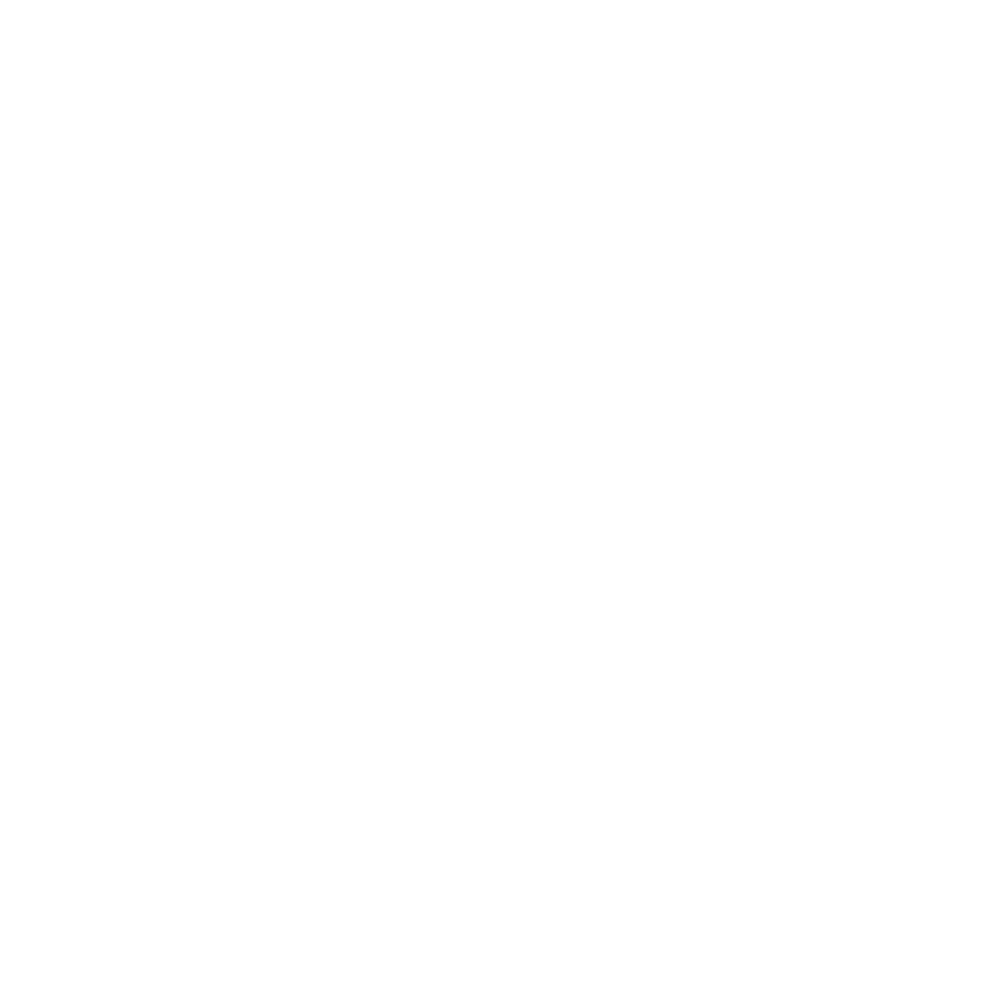# Statistics for Analytical Chemists

1 Day

## An introductory course to the fundamental of statistics, relevant to all professionals in the analytical sciences, life sciences and related fields.

This course comprehensively covers the basic concepts and methods of statistics with applications in the field of analytical sciences. During this course, we will demonstrate methods of exploring, organising, and presenting data, and introduce the fundamentals of probability.

Explore the foundations of statistical inference, including the concepts of parameters and estimates. Find out more about the use of statistical measures, confidence intervals, and hypothesis tests.

• Quantifying Errors
• Normal (Gaussian) Distribution
• Significance Tests
• Statistical Measures
• Confidence Limits
• Analysis of Variance
• Regression

### Introduction

• Analytical problems
• Errors in quantitative analysis
• Random and systematic errors
• Accuracy, repeatability, reproducibility
• Standard reference materials

### Statistical Measures

• Mean and standard deviation
• Variance and coefficient of variation

### Normal (Gaussian) Distribution

• Sampling distributions
• Confidence limits
• Significant figures
• Propagation of errors

### Significance Tests

• Null hypothesis, type I and type II errors
• Comparison of